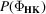International Tables for Crystallography (2012). Vol. F, ch. 16.1, pp. 413-432   | 1 | 2 | https://doi.org/10.1107/97809553602060000850

## Contents

• 16.1. Ab initio phasing  (pp. 413-432)
• 16.1.1. Introduction  (pp. 413-415) | html | pdf |
• 16.1.1.1. Data resolution  (pp. 413-414) | html | pdf |
• 16.1.1.2. Data completeness  (p. 414) | html | pdf |
• 16.1.1.3. Summary  (pp. 414-415) | html | pdf |
• 16.1.2. Normalized structure-factor magnitudes  (pp. 415-416) | html | pdf |
• 16.1.2.1. SIR differences  (p. 416) | html | pdf |
• 16.1.2.2. SAD differences  (p. 416) | html | pdf |
• 16.1.2.3. Difference intensities and direct methods  (p. 416) | html | pdf |
• 16.1.3. Starting the phasing process  (pp. 416-417) | html | pdf |
• 16.1.3.1. Structure invariants  (pp. 416-417) | html | pdf |
• 16.1.3.2. Multisolution' methods and trial structures  (p. 417) | html | pdf |
• 16.1.4. Reciprocal-space phase refinement or expansion ( shaking )  (pp. 417-418) | html | pdf |
• 16.1.4.1. The tangent formula  (p. 417) | html | pdf |
• 16.1.4.2. The minimal function  (pp. 417-418) | html | pdf |
• 16.1.4.3. Parameter shift  (p. 418) | html | pdf |
• 16.1.5. Real-space constraints ( baking )  (pp. 418-419) | html | pdf |
• 16.1.5.1. Simple peak picking  (p. 418) | html | pdf |
• 16.1.5.2. Iterative peaklist optimization  (pp. 418-419) | html | pdf |
• 16.1.5.3. Random omit maps  (p. 419) | html | pdf |
• 16.1.6. Fourier refinement  (p. 419) | html | pdf |
• 16.1.7. Resolution enhancement: the free lunch' algorithm  (p. 419) | html | pdf |
• 16.1.8. Utilizing Pattersons for better starts  (pp. 419-420) | html | pdf |
• 16.1.9. Shake-and-Bake : an analysis of a dual-space method in action  (pp. 420-422) | html | pdf |
• 16.1.9.1. Flowchart and program comparison  (pp. 420-421) | html | pdf |
• 16.1.9.2. Parameters and procedures  (p. 421) | html | pdf |
• 16.1.9.3. Recognizing solutions  (pp. 421-422) | html | pdf |
• 16.1.10. Applying dual-space programs successfully  (pp. 422-425) | html | pdf |
• 16.1.10.1. Avoiding false minima  (pp. 422-423) | html | pdf |
• 16.1.10.2. Choosing a refinement strategy  (pp. 423-424) | html | pdf |
• 16.1.10.3. Expansion to P 1  (pp. 424-425) | html | pdf |
• 16.1.10.4. Substructure applications  (p. 425) | html | pdf |
• 16.1.11. Substructure solution for native sulfurs and halide soaks  (pp. 425-426) | html | pdf |
• 16.1.12. Computer programs for dual-space phasing  (pp. 426-429) | html | pdf |
• 16.1.12.1. ACORN   (pp. 426-427) | html | pdf |
• 16.1.12.2. IL MILIONE   (pp. 427-428) | html | pdf |
• 16.1.12.3. SHELX   (p. 428) | html | pdf |
• 16.1.12.4. SnB and BnP   (p. 428) | html | pdf |
• 16.1.12.5. HySS   (p. 428) | html | pdf |
• 16.1.12.6. SUPERFLIP : charge flipping  (pp. 428-429) | html | pdf |
• 16.1.12.7. CRUNCH2 – Karle–Hauptman determinants  (p. 429) | html | pdf |
• 16.1.13. Conclusions and the grand challenge  (p. 429) | html | pdf |
• References | html | pdf |
• Figures
• Fig. 16.1.1.1. Averaged squared normalized structure-factor amplitudes over 700 protein structures with standard deviations calculated from the population of individual | E | 2 profiles (from Morris & Bricogne, 2003)  (p. 413) | html | pdf |
• Fig. 16.1.1.2. ( a ) Mean phase error as a function of resolution for the two independent ab initio SHELXD solutions of the previously unsolved protein hirustasin  (p. 414) | html | pdf |
• Fig. 16.1.3.1. The conditional probability distribution,, of the three-phase structure invariants,, having associated parameters A HK with values of 0, 1, 2, 4 and 6  (p. 416) | html | pdf |
• Fig. 16.1.9.1. A flowchart for the Shake-and-Bake procedure, which is implemented in both SnB and SHELXD   (p. 420) | html | pdf |
• Fig. 16.1.9.2. A histogram of figure-of-merit values (minimal function) for 378 scorpion toxin II trials  (p. 421) | html | pdf |
• Fig. 16.1.9.3. Tracing the history of a solution and a nonsolution trial for scorpion toxin II as a function of Shake-and-Bake cycle  (p. 422) | html | pdf |
• Fig. 16.1.10.1. Success rates for triclinic lysozyme are strongly influenced by the size of the parameter-shift angle  (p. 423) | html | pdf |
• Fig. 16.1.10.2. ( a ) Success rates and ( b ) cost effectiveness for several dual-space strategies as applied to a 148-atom P 1 structure  (p. 424) | html | pdf |
• Fig. 16.1.10.3. Success rates for the 317-atomstructure of gramicidin A  (p. 424) | html | pdf |
• Fig. 16.1.11.1. Relative occupancy against peak number for SHELXD substructure solutions of elastase  (p. 426) | html | pdf |
• Tables
• Table 16.1.1.1. Success rates for three P 1 structures illustrate the importance of using complete data to the highest possible resolution  (p. 415) | html | pdf |
• Table 16.1.1.2. Improving success rates by `completing' the vancomycin data  (p. 415) | html | pdf |
• Table 16.1.2.1. Theoretical values pertaining to's  (p. 415) | html | pdf |
• Table 16.1.8.1. Overall success rates for full structure solution for hirustasin using different two-atom search vectors chosen from the Patterson peak list  (p. 420) | html | pdf |
• Table 16.1.9.1. Recommended parameter values for the SnB  program  (p. 421) | html | pdf |
• Table 16.1.10.1. Some large structures solved by the Shake-and-Bake method  (p. 423) | html | pdf |